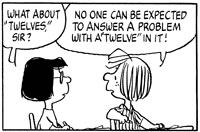## Thursday, June 14, 2012

### put in one's placeConsider, if you will, the question "What is the last digit of 4316 * 12  + 511?"

One way to quickly answer "what is the last digit?" questions like this is to realize that you don't need to do the full calculation - only the last digits of each number in the problem contribute to the last digit in the answer. So, you just need to calculate 6 * 2 + 1 and look at the last digit of that, which is 3.

When can we use this short-cut? With impunity when only multiplication and addition of positive Integers are involved - here only digits-in-the-ones-place of the inputs affect the digit-in-the-ones-place of the output. If you throw multiplication's tricky partner division into the mix, then I think that all bets are off (digits in all places affect the ones position in the result when you divide). However, we can still proceed with caution when  subtraction and negative Integers are in play: if we get a negative along the way while we are looking at the ones place, we need to pause and see if we should have "borrowed" from the tens place that we were ignoring.

For example, what is the last digit of (91536 - 648) * 12? Ignoring everything except the last digits in the original problem we have (6 - 8) * 2 = ( -2 ) * 2. At this point, we need to stop and realize that instead of keeping the -2 we should have borrowed from the tens position of  91536, giving us an 8 in the ones position, so we should have (8) * 2 = 16, so the last digit of (91536 - 648) * 12 is 6.

PS: The rest of the Peanuts cartoon featuring Peppermint's inventive rules for arithmetic can be found here.